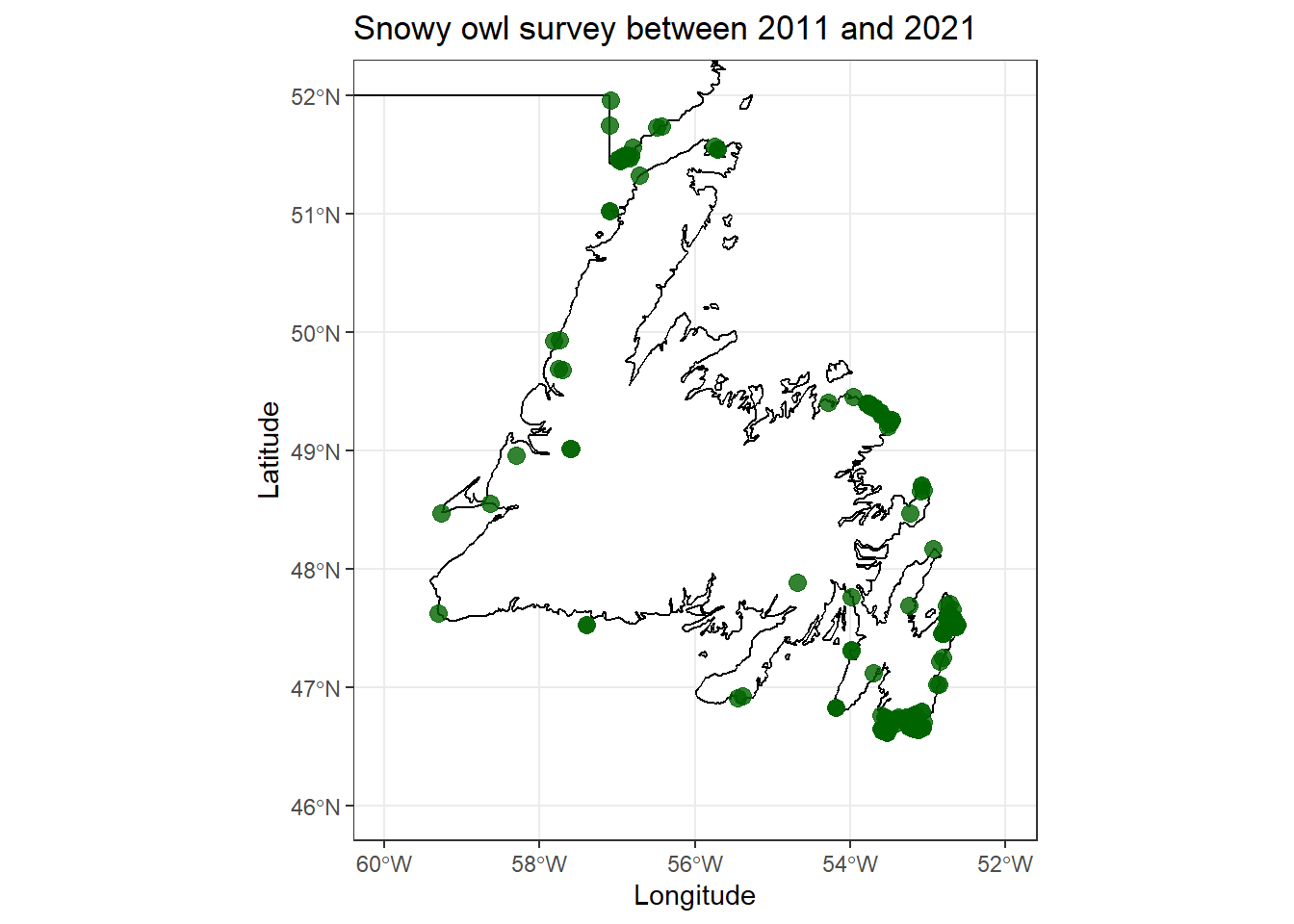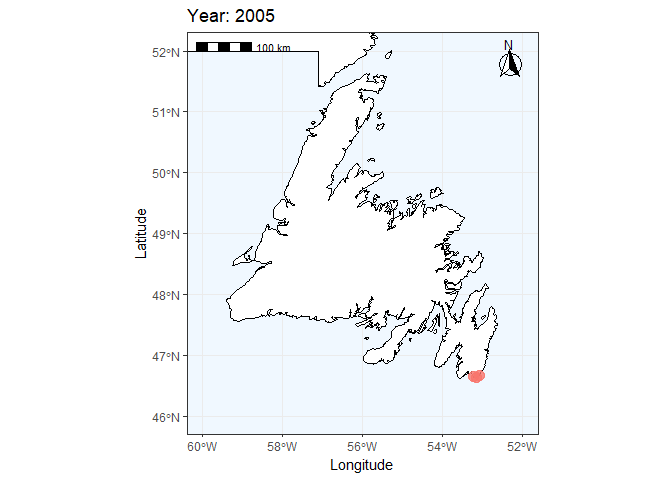# 5 Create GIF animation

## 5.1 Create static map

Let’s use the previous script map from NL with zoom on Newfoundland island. Before running this, you need to have created map_nl, see Import background basemap Import background basemap

Add the data on the map

• `geom_point` function to add the data with x (longitude) and y (latitude) axis. The projection of the data needs to be in the same projection than the background map.
• `coord_sf` allow here to set manually the zoom on specific area based on x and y axis limits.
``````ggplot() +
geom_sf(data = map_nl, colour = "black", fill = "white") +
geom_sf() +
# We can add an ENTIRELY new data set inside a geom
geom_point(data = birdsurvey,
aes(x = decimalLongitude,
y = decimalLatitude),
alpha = 0.1,
colour = "blue") +
labs(
title = "Bird survey between 2002 and 2021"
) +
xlab("Longitude")+ ylab("Latitude") +
# Zoom in to a specific view
coord_sf(xlim = c(-60, -52),
ylim = c(46, 52))``````## 5.2 Subset species and period

Bubo scandiacus

``````Bubo <- subset(birdsurvey, species == 'Bubo scandiacus')
Bubo_2011_2021 <- subset(Bubo, year >= 2011 & year <= 2021)``````
``````### Plot Bubo distribution only
ggplot() +
geom_sf(data = map_nl, colour = "black", fill = "white") +
geom_sf() +
# We can add an ENTIRELY new data set inside a geom
geom_point(data = Bubo_2011_2021,
aes(x = decimalLongitude,
y = decimalLatitude),
alpha = 0.8,
size = 3,
colour = "darkgreen") +
labs(
title = "Snowy owl survey between 2011 and 2021"
) +
xlab("Longitude")+ ylab("Latitude") +
# Zoom in to a specific view
coord_sf(xlim = c(-60, -52),
ylim = c(46, 52))+
theme_bw()``````## 5.3 Create GIF by year

Set the map for the GIF Here the main difference with a static plot is the function `transition_time` from the package `gganimate`. This function allow to set which column we want to use to get one image by period (here, by year).

``````### Create a ggplot + add specific gganimate function = transition_time
plot1 <- ggplot()  +
geom_sf(data = map_nl, colour = "black", fill = "white")  +
# We can add an ENTIRELY new data set inside a geom
geom_point(data = Bubo,
aes(x = decimalLongitude,
y = decimalLatitude,
color = as.factor(year)),
alpha = 0.8,
size = 3.5)  +
transition_time(year) + #### this will allow us to get one image of data by year
labs(
title = "Bird survey between 2002 and 2021"
) +
xlab("Longitude")+ ylab("Latitude") +
# Zoom in to a specific view
coord_sf(xlim = c(-60, -52),
ylim = c(46, 52)) +
labs(title = "Year: {frame_time}") ``````

## 5.4 Add scale bar and north arrow

A map always needs scale and north arrow. Here we separately set the function to add the two layers to the map previously created before (plot1)

``````##### Add scale bar and north arrow
plot1 <- plot1 +
annotation_scale(location = "tl", width_hint = 0.3) +
annotation_north_arrow(location = "tr", which_north = "true",
height = unit(1, "cm"), width = unit(1, "cm"),
• The function `animate` from the package `gganimate` is the function use to create the GIF output. There is different options to set. Here we use only the options: `nframes` and `duration` to define the number of frames per second (one frame per year (10 years of survey) for 15 seconds of animation).
``````######  This part will create the gif